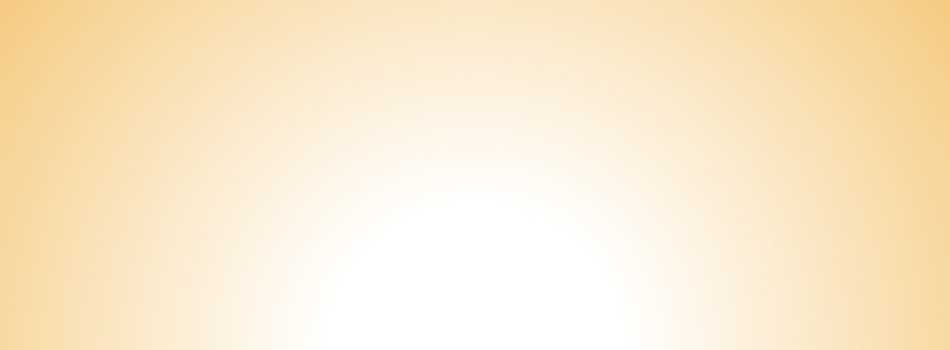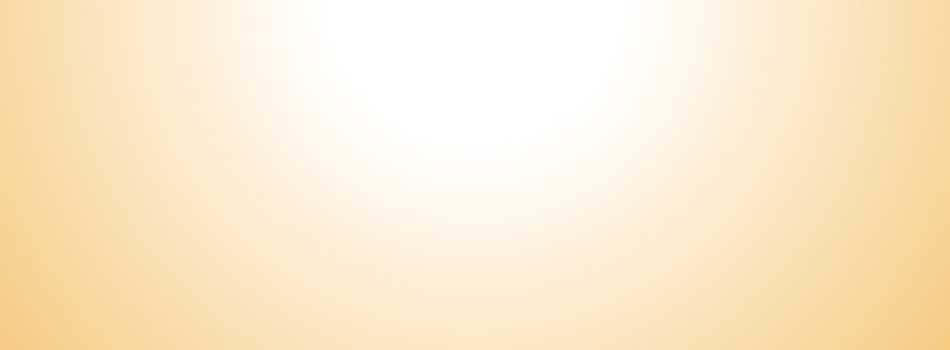The Large Studio Kit and Upgrade

The Large Studio Kit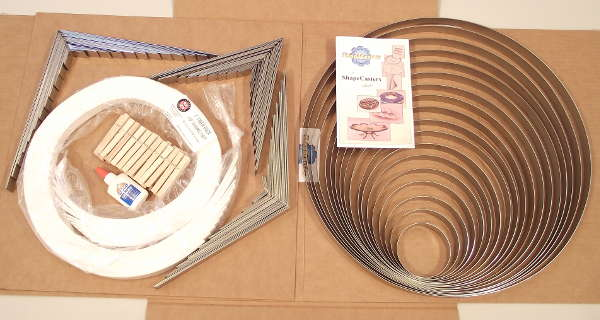This kit is so big, we had trouble fitting it into our photo setup! It's our largest kit so far. The Large Studio Kit is made for the artist with plenty of kiln space who wants a full range of shapes to work with, or as a backbone set for large working studios.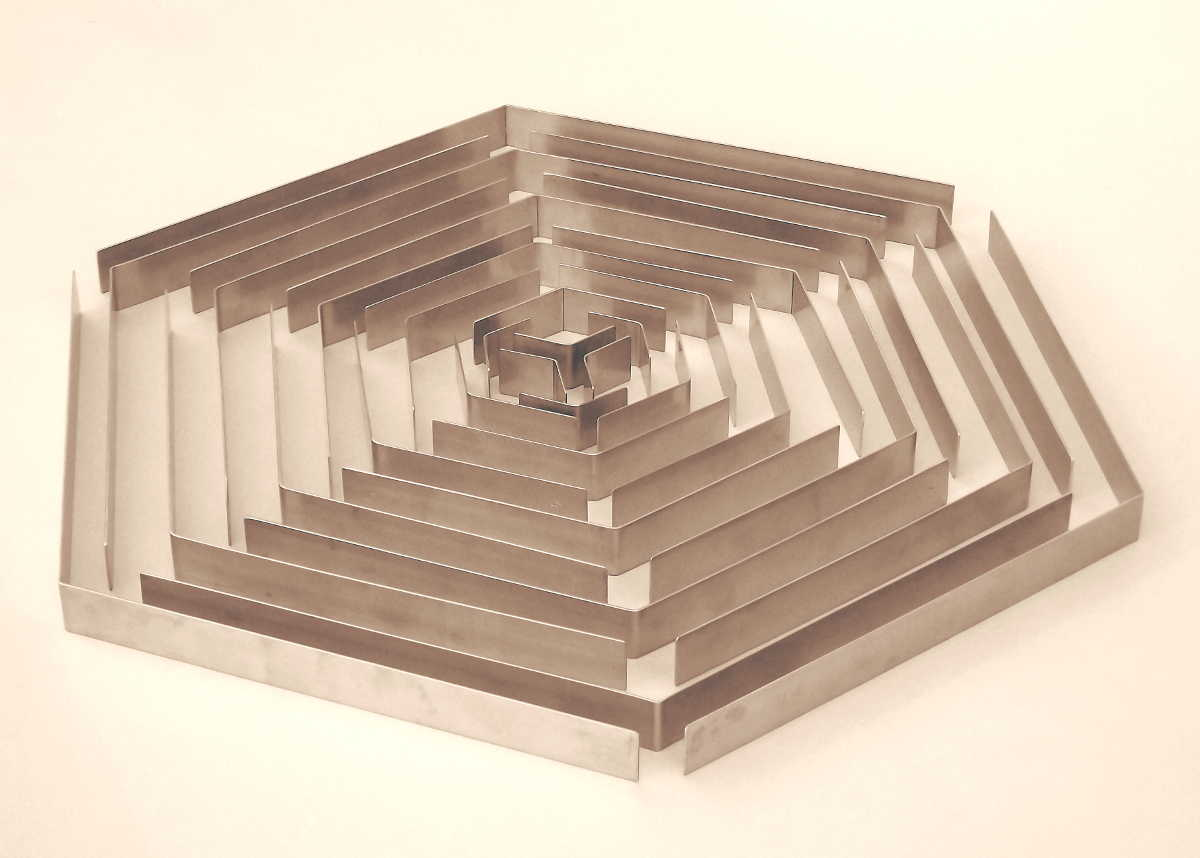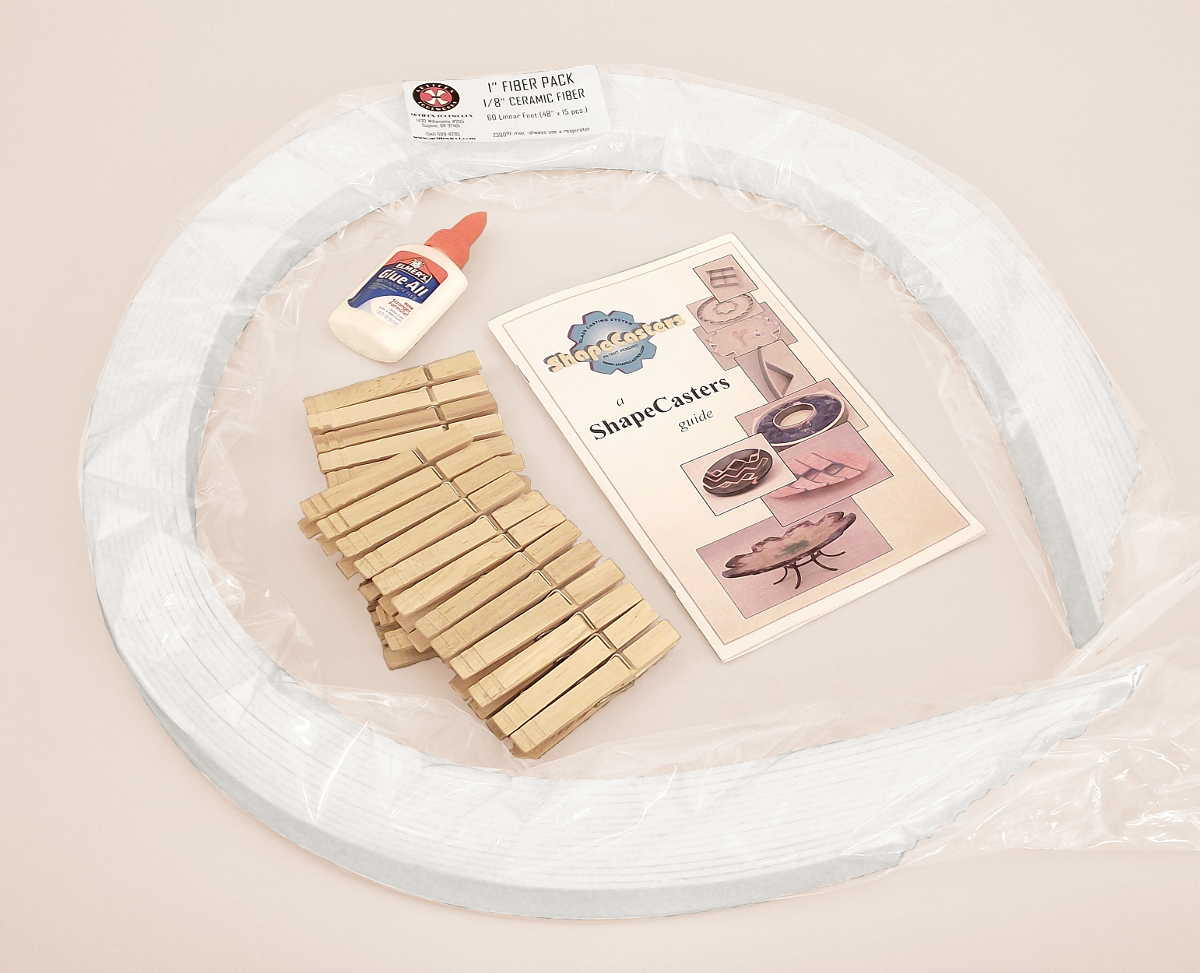Contents:
Circles
1 ea. #3 Circle
1 ea. #4 Circle
1 ea. #5 Circle
1 ea. #6 Circle
1 ea. #7 Circle
1 ea. #8 Circle
1 ea. #9 Circle
1 ea. #10 Circle
1 ea. #11 Circle
1 ea. #12 Circle
1 ea. #13 Circle
1 ea. #14 Circle
1 ea. #15 Circle
1 ea. #16 Circle
1 ea. #17 Circle
1 ea. #18 Circle
1 ea. #19 Circle
1 ea. #20 Circle
Tri Angles
3 ea. #1 Tri equal leg angle
3 ea. #2 Tri equal leg angle
3 ea. #3 Tri equal leg angle
3 ea. #4 Tri equal leg angle
3 ea. #5 Tri equal leg angle
3 ea. #6 Tri equal leg angle
3 ea. #7 Tri equal leg angle
3 ea. #8 Tri equal leg angle
3 ea. #9 Tri equal leg angle
3 ea. #10 Tri equal leg angle
4 ea. #1 Quad equal leg angle
2 ea. #2 Quad equal leg angle
2 ea. #3 Quad equal leg angle
2 ea. #4 Quad equal leg angle
2 ea. #5 Quad equal leg angle
2 ea. #6 Quad equal leg angle
2 ea. #7 Quad equal leg angle
2 ea. #8 Quad equal leg angle
2 ea. #9 Quad equal leg angle
2 ea. #10 Quad equal leg angle
2 ea. #11 Quad equal leg angle
2 ea. #12 Quad equal leg angle
Hex Angles
6 ea. #1 Hex equal leg angle
3 ea. #2 Hex equal leg angle
3 ea. #3 Hex equal leg angle
3 ea. #4 Hex equal leg angle
3 ea. #5 Hex equal leg angle
3 ea. #6 Hex equal leg angle
3 ea. #7 Hex equal leg angle
3 ea. #8 Hex equal leg angle
3 ea. #9 Hex equal leg angle
3 ea. #10 Hex equal leg angle
extras
1 ea. 1" fiberPak (60 linear feet)
24 ea. spring clips
1 ea. glue bottle
1 ea. ShapeCaster Guide

107 ShapeCasters and a Goodie Pack, retail value: \$1166.25, a 39% savings

 SC_LSK Large Studio Kit (108 pcs) \$ 700.00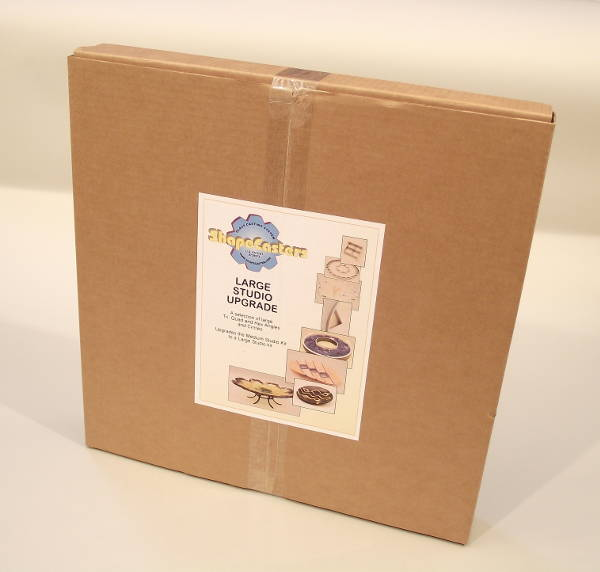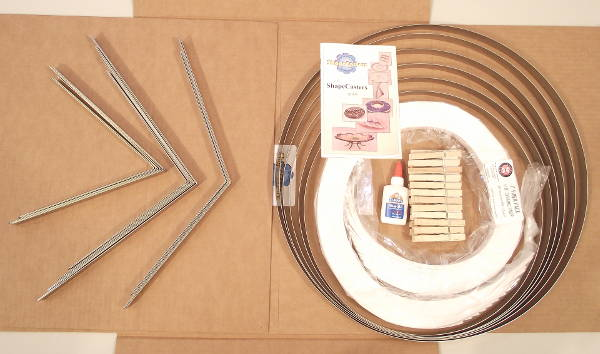Already have a Medium Studio Kit, or just want the big parts? The Large Studio Upgrade lets you do that. It's 26 Shapecasters are just what you need to make a variety of large shapes. And, of course, you get another Goodie Pack...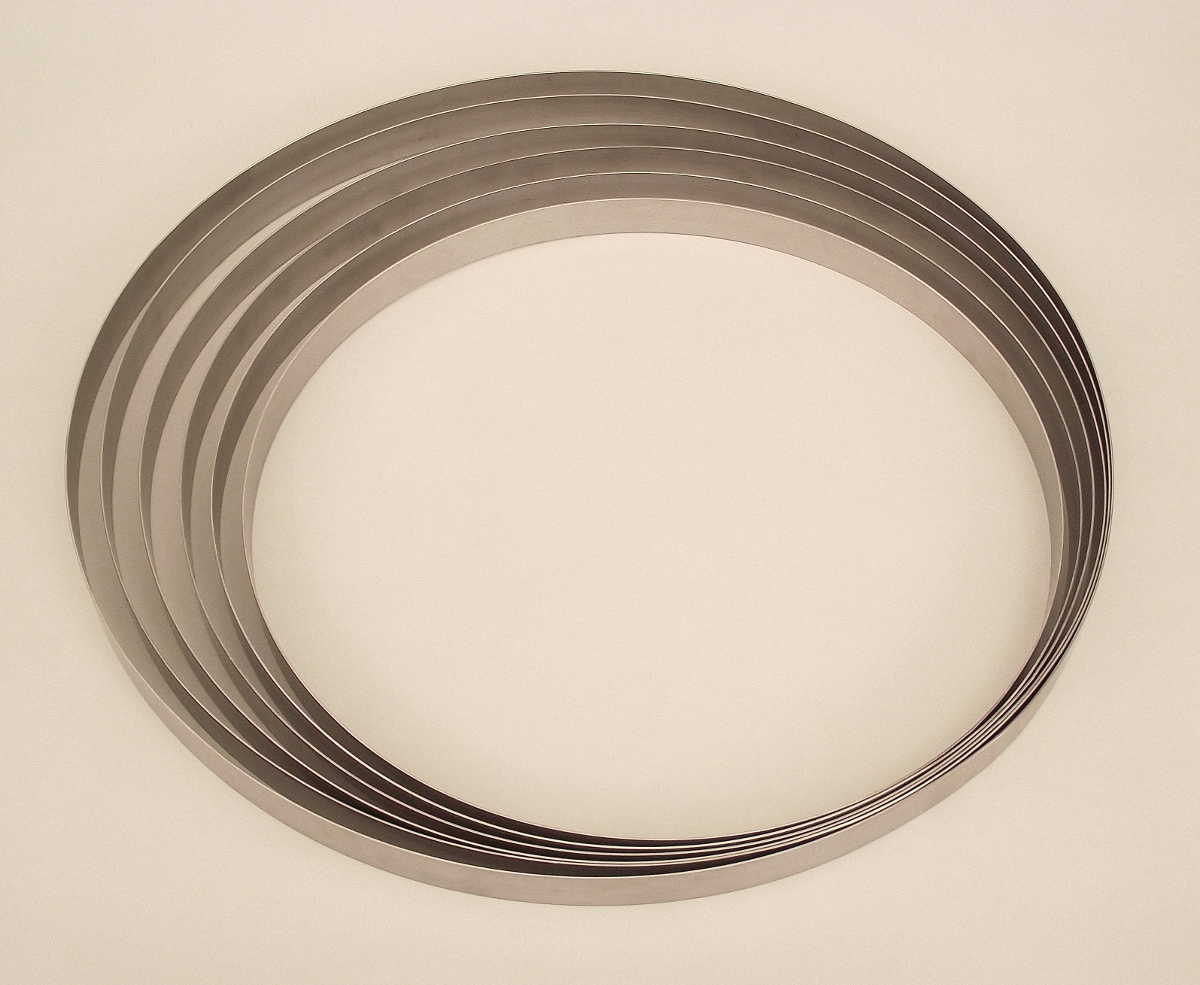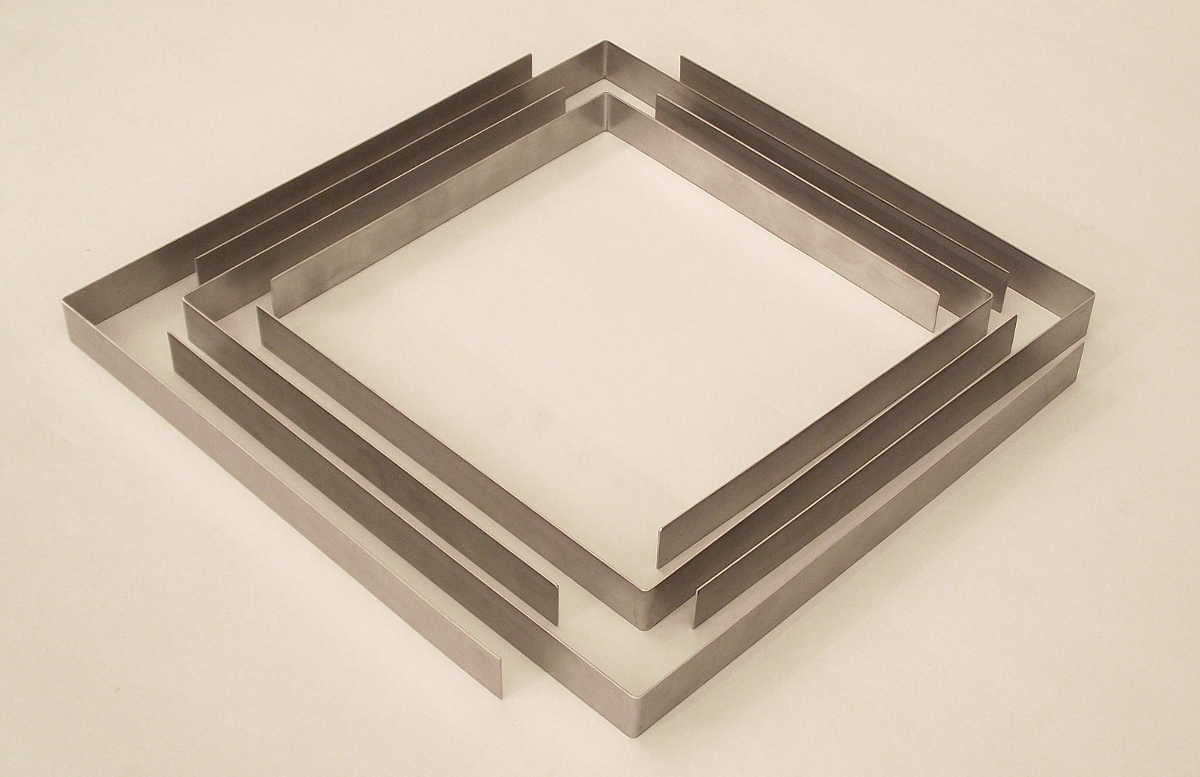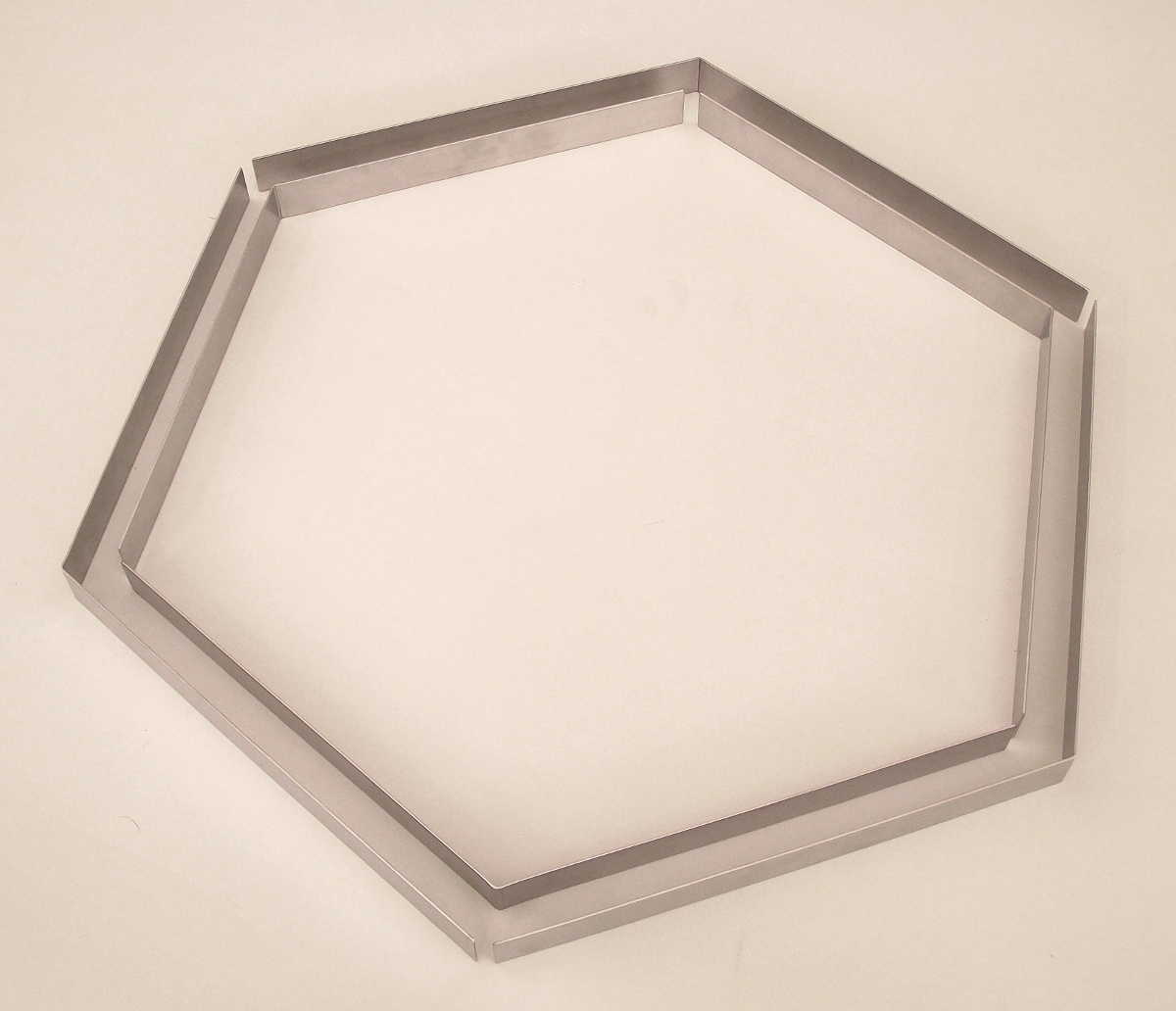Contents:
Circles
1 ea. #15 Circle
1 ea. #16 Circle
1 ea. #17 Circle
1 ea. #18 Circle
1 ea. #19 Circle
1 ea. #20 Circle
Tri Angles
3 ea. #9 Tri equal leg angle
3 ea. #10 Tri equal leg angle
2 ea. #9 Quad equal leg angle
2 ea. #10 Quad equal leg angle
2 ea. #11 Quad equal leg angle
2 ea. #12 Quad equal leg angle
Hex Angles
3 ea. #9 Hex equal leg angle
3 ea. #10 Hex equal leg angle
extras
1 ea. 1" fiberPak (60 linear feet)
24 ea. spring clips
1 ea. glue bottle
1 ea. ShapeCaster Guide

26 ShapeCasters and a Goodie Pack, retail value: \$477.45, a 26% savings

 SC_LSUG Large Studio Upgrade (27 pcs) \$ 350.00